Reconstruction of the 2014 Ackling Dyke formation 1.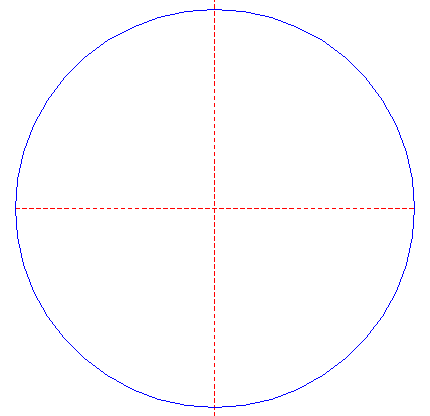Draw a circle. Draw and extend the horizontal and vertical centerlines. 2.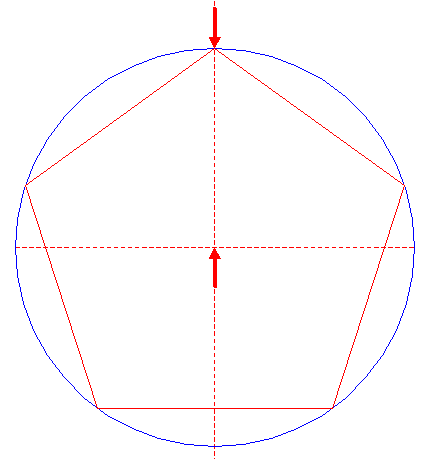Construct the inscribed pentagon (regular 5-sided polygon) of circle 1, pointing up. 3.Construct the inscribed circle of pentagon 2. 4.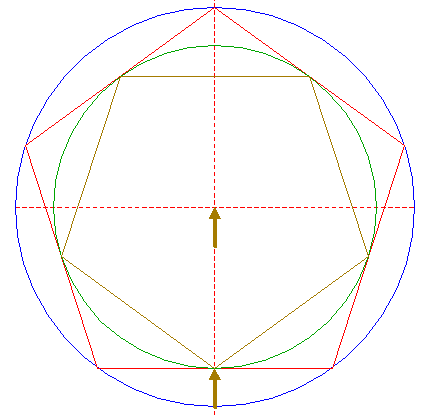Construct the inscribed pentagon of circle 3, pointing down. 5.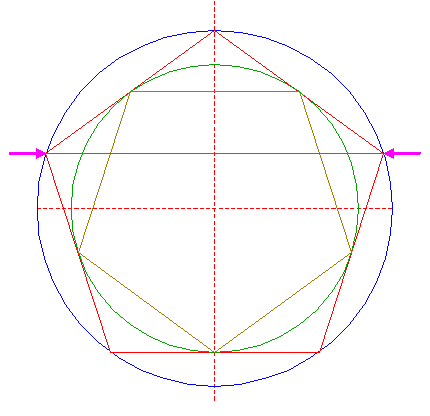Draw the diagonal between the leftmost and rightmost angular points of pentagon 2. 6.Construct a circle concentric to circle 1, tangent to diagonal 5. 7.Copy circle 6 five times, to the angular points of pentagon 2. 8.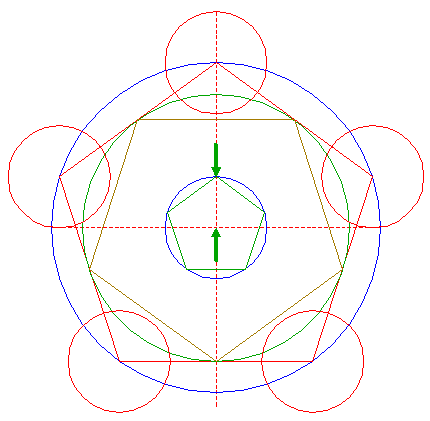Construct the inscribed pentagon of circle 6, pointing up. 9.Draw the diagonal between the leftmost and rightmost angular points of pentagon 8. 10.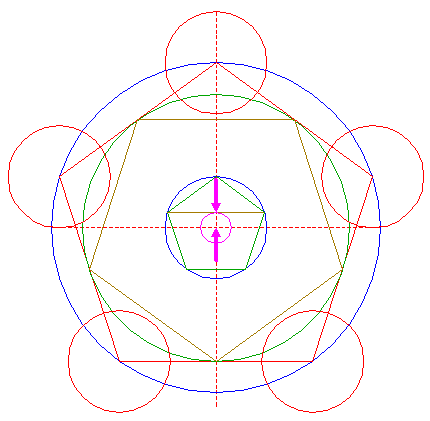Construct a circle concentric to circle 1, tangent to diagonal 9. 11.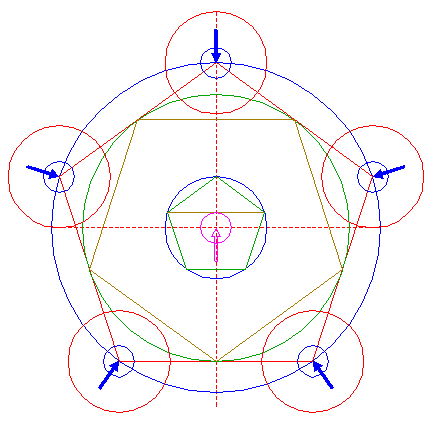Copy circle 10 five times, to the centers of circles 7. 12.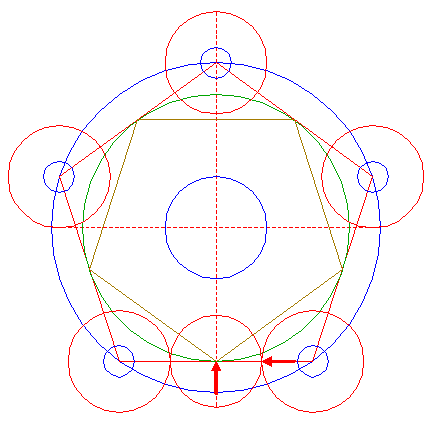Construct a circle centered at the lower angular point of pentagon 4, tangent to the lower righthand circle 7 at the lefthand side. 13.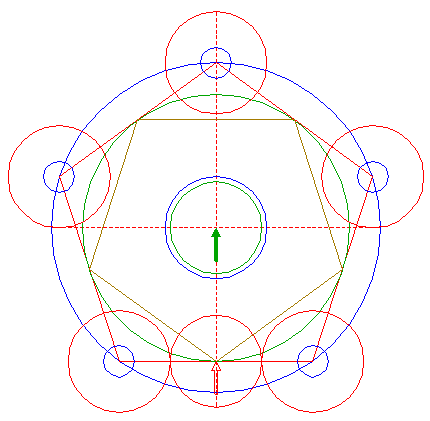Copy circle 12 to the center of circle 1. 14.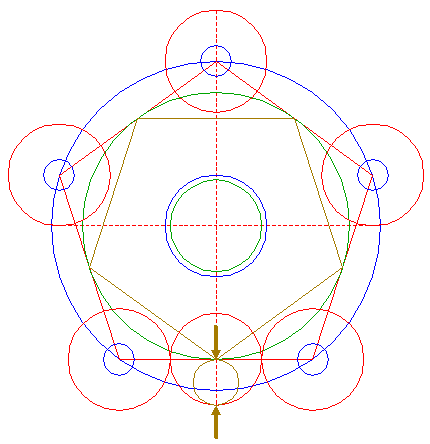Construct a "two-points" circle (defined by the two end-points of a centerline) between the center of circle 12 and its lower intersection with the vertical centerline. 15.Copy circle 14 to the center of circle 1. 16.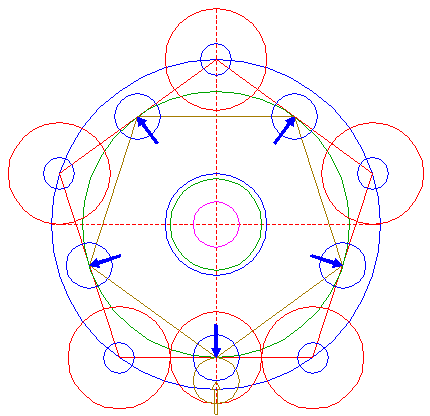Copy circle 14 five times, to the angular points of pentagon 4. 17.Draw the five rays of pentagon 4, from the center of circle 1 to its angular points. 18.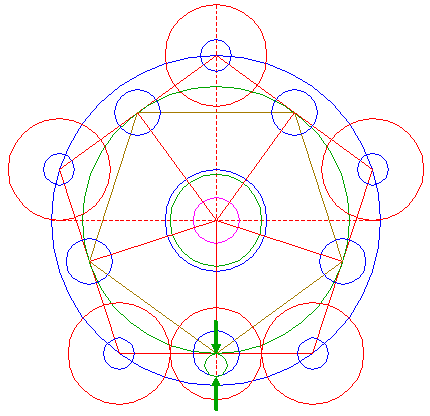Construct a "two-points" circle between the center of the lower circle 16 and its lower intersection with the vertical centerline. 19.Copy circle 18 five times, to the intersections of circle 13 and rays 17. 20.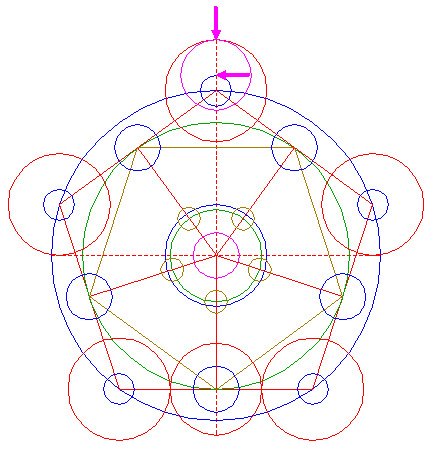Construct a circle centered at the upper intersection of upper circle 11 and the vertical centerline, tangent to upper circle 7 at the upper side. 21.Repeat step 20 four times, with respect to the other circles 11 and 7. 22.Draw two connecting lines, between the center of lower circle 16 and the centers of leftmost and rightmost circles 19. 23.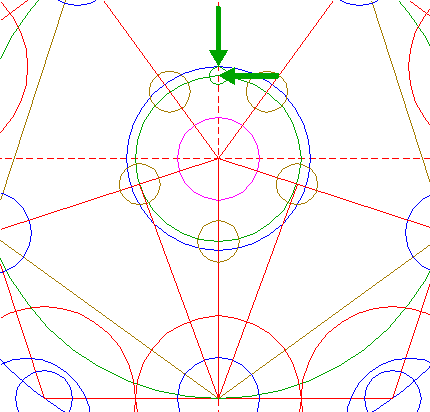Construct a circle centered at the upper intersection of circle 13 and the vertical centerline, tangent to circle 6 at the upper side. 24.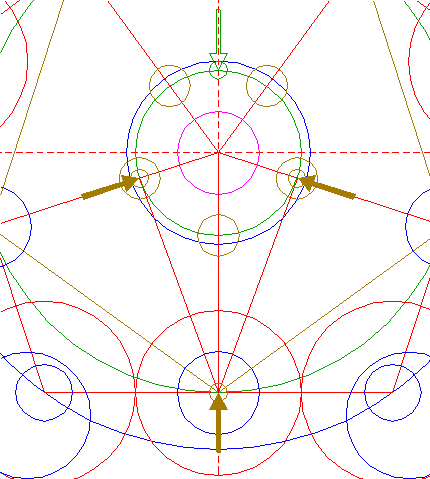Copy circle 23 three times, to the end-points of lines 22. 25.Construct two lines, parallel to lines 22 at the inner sides, tangent to circles 24, as shown. 26.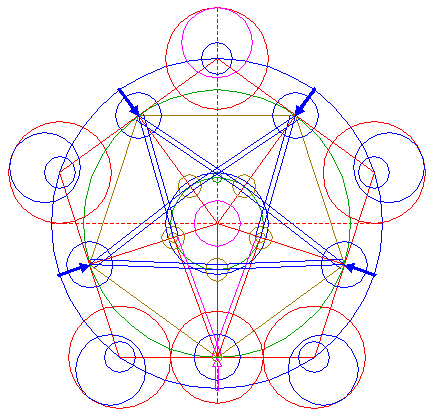Repeat steps 22 and 25 four times, with respect to the other circles 16 and 19. 27.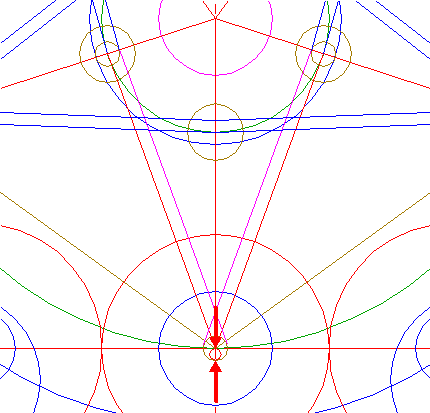Construct a "two-points" circle between the center of the lower circle 24 and its lower intersection with the vertical centerline. 28.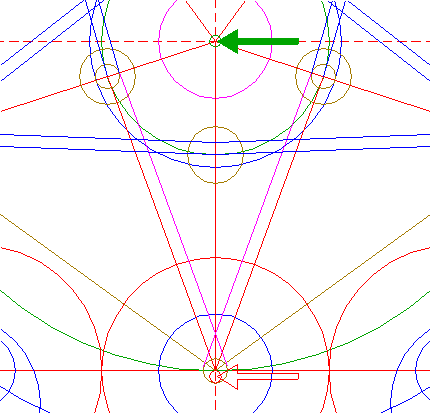Copy circle 27 to the center of circle 1. 29.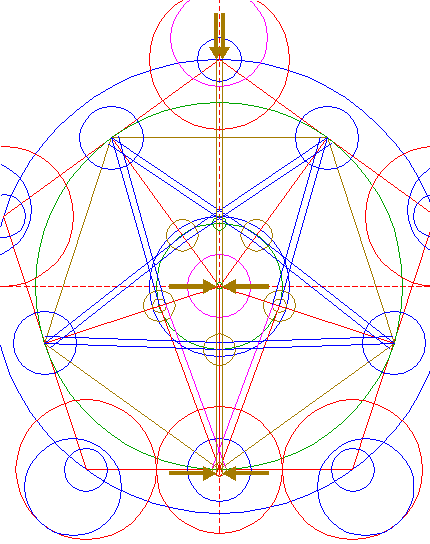Construct two parallel lines, tangent to circles 27 and 28 at both sides, and extend these lines upward upto circle 1. See detail.30.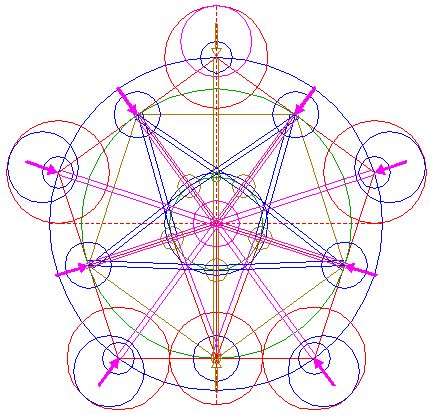Repeat step 29 four times, with respect to the other circles 11 and 16, as shown. 31.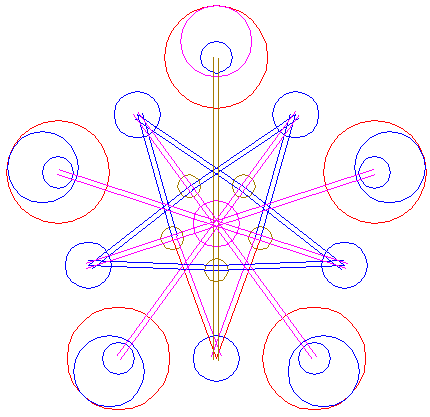Circles 7, 11, 15, 16, 19, 20 and 21, and lines 22, 25, 26, 29 and 30, are used for the final reconstruction. 32.Remove all parts not visible within the formation itself. 33.Colour all areas corresponding to standing... 34....or to flattened crop, and finish the reconstruction of the 2014 Ackling Dyke formation. 35.The final result, matched with the aerial image.
 Copyright © 2014, Zef Damen, The Netherlands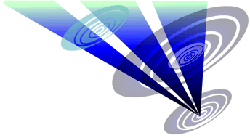BACKMark Fussell & Stuart Dike